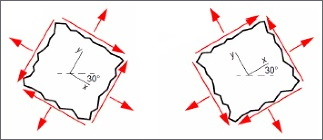# Examination with random values

This is an example of a specific examination conducted at KTH. In this examination solution, examples are given of good ways to vary tasks about calculations without affecting the students' ability to show intended learning outcomes at the same level. The methods to vary tasks have been developed with the challenge in mind of how to determine whether students use the same values due to a typo or if it is due to cheating.

## The exam content

The exam was conducted remotely and consisted of six assignments where the students in all assignments could show knowledge corresponding to level E-A. The assessment was made on the basis of an overall picture of what the student has achieved on all assignments. In order for the students to have the opportunity to prioritize their time, the exam began with an overview of what the exam assignments would deal with. Then the students got access to two assignments at a time, paired together according to how much time they were expected to take to solve. A Canvas quiz distributed the questions and the students submitted the answers before receiving the next pair of assignments. When the entire exam was completed, the students scanned their solutions and submitted them to a Canvas assignment so that assessment could take place in Speedgrader.

## Examples of variations of a question

Question: Determine the length of the hypotenuse in the green triangle.

A = 67
B = (your date of birth + 100) / 2
C = 37 (a by Canvas picked random value between 31 and 49)

The students could also be assigned the following variation of the question from an item bank,

• The wording was the same but the annotation of the image (A, B, C) was changed, see change in the red rings.
• The wording was the same but the picture was changed, see the green field.

In each assignment, the student had to enter values ​​based on their personal identity number. Additionally, the assignments had random values created by the quiz's built-in features. The questions were selected from a Canvas item bank, where each question actually consisted of a set of questions with small variations in the design, and random values using the Canvas' question type "formula question".Examples of the images for two different versions of a question. The wording with each image was the same.

Each student had an equal opportunity to show the intended learning outcomes even though they all had different exams. The students were informed in advance that the examinations' assignments would differ from student to student, that values would be randomized or based on social security numbers. As it is not relevant for the students' preparation for the examination, they were not specifically informed that the questions could also vary in small details.

Because each assignment differed from student to student, a higher reliability of the examination was achieved. When reviewing, it is more difficult to dismiss plagiarized information (where students "happen" to use the same value) as a typo, as there are several values that may "accidentally" coincide. To simplify the assessment work, a Matlab code was written so that when the student's personal identity number and the students randomized values were entered, the solution for all tasks was shown based on the correct values for the student.

## Experiences of monitoring the exam with Zoom

During the examination, students are allowed to formula collections and calculators, but preferably not literature with calculation examples, and absolutely no contact with other students. The course was therefore examined with a five-hour exam in Zoom with three scheduled breaks and 24 students for each room and exam invigilator. As this was a re-examination, only half of the students showed up, which made the Zoom monitoring unnecessarily resource-intensive in relation to advantages of monitoring.

In order to balance the advantages and use of resources in future remote examinations where an identity check is to be carried out, it is recommended that the zoom presence  solution be used instead.